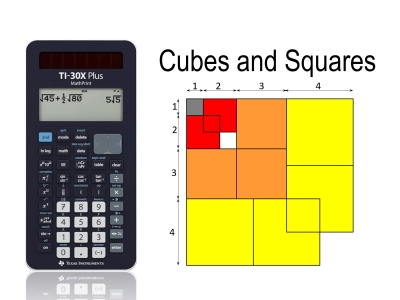# Activities

•• ##### AuthorAust Senior

25 Minutes

## Cubes and Squares#### Activity Overview

What is the relationship between the sums of whole numbers and the sums of cubed numbers? The PowerPoint slide show makes this connection in a very powerful visual way. Students then use their calculator to explore and finally prove the result by induction.

#### Objectives

The powerful visuals in this activity on their own can help students determine a formula for the sum of cubed numbers, however, it is proof that shows the result is true for all positive whole numbers. The proof is relatively easy considering the apparent complexity of the equation (degree 4 polynomial). A little intelligent use of common factors makes the proof much easier.

#### Vocabulary

• Induction
• Proof
• Whole number sums
• Summation